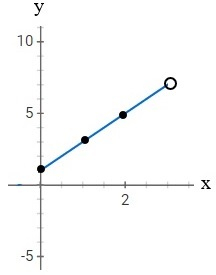# Which 12

Which table shows a proportional relationship between x and y?

A)
x 3 9 10 15
y 1 3 4 5

B)
x 2 3 5 6
y 3 4 7 9

C)
x 1 5 8 10
y 15 75 120 150

D)
x 4 6 8 10
y 6 8 10 12

Result

A=

B=

C=

D=

### Step-by-step explanation:Did you find an error or inaccuracy? Feel free to write us. Thank you!

Tips for related online calculators
The line slope calculator is helpful for basic calculations in analytic geometry. The coordinates of two points in the plane calculate slope, normal and parametric line equation(s), slope, directional angle, direction vector, the length of the segment, intersections of the coordinate axes, etc.